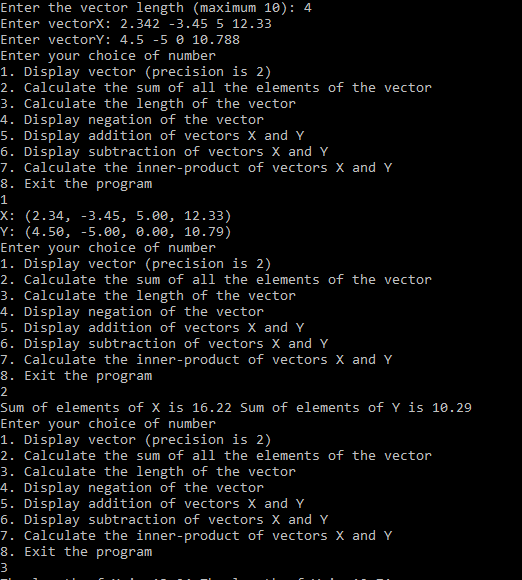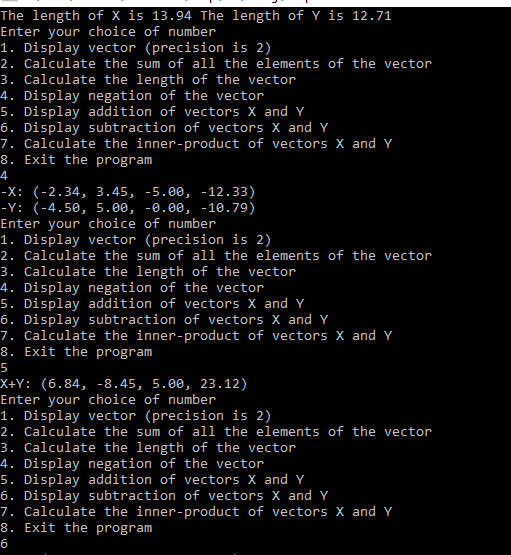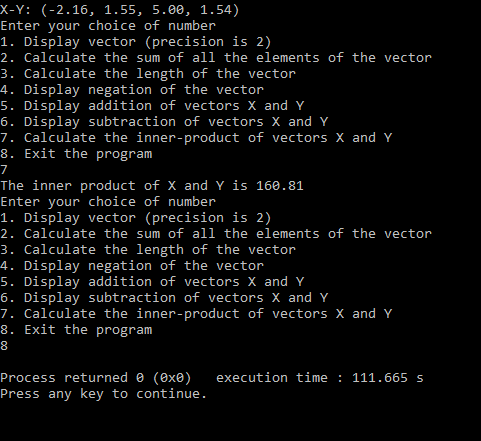# Answered Essay: please I need help with this c programming pro

please I need help with this c programming pro

Project Description:

In this project, you will develop a calculator for vectors. Your program will .ask the user

to read in two vectors X and Y. The user will first enter the length of each vector and followed by each element of the vector. The maximum vector length is 10.

Enter the vector length (maximum 10): 4

EntervectorX: 2.342-3.45 5 12.33

Enter vector Y: 4.5 -5 0 10.788

Then your program will display a menu of options and ask the user to choose one of the options repeatedly until the user chooses the exit (option 8). Below is a list of options and their descriptions:

 Option Description 1 Display vector (precision is 2) 2 Calculate the sum of all the elements of the vector 3 Calculate the length of the vector 4 Display negation of the vector 5 Display addition of vectors X and Y 6 Display subtraction of vectors X and Y 7 Calculate the inner-oroduct of vectors X and Y 8 Exit the prooram

Option 1: ·

This option simply displays both vectors in the following required format with precision 2. Separate the elements by commas followed by a single space.

Sample output:

X: (2.34, -3.45, 5.00, 12.33)

Y: (4.50, -5.00, 0.00, 10.79)

Option 2:

This option calculates and displays the sum of all the elements of each vector. Sample output:

Sum of elements of Xis 16.22 Sum of elements of Y is 10.29

Option3:

This option calculates and displays the length of each vector, i.e., square root of the sum of

·square of each element in the vector.

The length of the vector X =.square root of (x1^2+x2^2+…+x^n)

Sample output:

The length of X is 13.94 The length of Y is 12.71.

Option 4

IfY = (y1,y2, Y3, ….Yn), then negation ofY gives (-y1, -Y2, -y3, …. -Yn). Sample output:

-X: (-2.34, 3.45, -5.00, -12.-33)

-Y: (-4.50, 5.00, 0.00, -10.79)

Option 5

This option calculates and displays X+Y, element by element. Sample output:

X+Y: (6.84, -8.45, 5.00, 23.12)

Option 6

This option calculates and displays X- Y, element by element. Sample output:

X-Y: (-2.16, 1.55, 5.00, 1.54)

Option 7

Tue inner product of two vectors X and Y is calculated as somme of i=1 to n xi, yi

Sample output:

The inner product of X and Y is 160.81

Option 8: Exit the program.

Error Checking:

Your program should check thoroughly for relevant errors. Upon encountering an error, the prograni should report an informative error message and handle the error accordingly. Certain errors are recoverable errors. Upon encountering such errors, the program should output a meaningful error message and continue the prograni, for example, if an invalid option is entered. Certain errors are fatal errors. Upon encountering fatal errors, the program should output a meaningful error message and exit. For example, if the length of the vector is not a positive integer or the length exceeds the maximum length.

Project Requirements:

Tue project should be well documented (comments) and properly indented. Title block is required (Name, project number, project description). Your code should be clear and well organized. Define of your own functions is highly recommended, but not required.

Submission Requirements:

When submitting your project, please submit an archive of your entire project directory. Use LIMITATIONS.txt to document anything that couldn’t be fully completed or debugged. No late submissions will be accepted.

**C Code:

#include <stdio.h>
#include <stdlib.h>
#include <math.h>
// Function to Display vector (precision is 2)
void displayVector(float a[], float b[], int length){
int i;
printf(“X: (“);
for(i = 0; i < length – 1; i++)
printf(“%.2f, “, a[i]);
printf(“%.2f”, a[length-1]);
printf(“)n”);
printf(“Y: (“);
for(i = 0; i < length – 1; i++)
printf(“%.2f, “, b[i]);
printf(“%.2f”, b[length-1]);
printf(“)n”);
}
// Function to Calculate the sum of all the elements of the vector
void sumOfVectors(float a[], float b[], int length){
float sumX = 0, sumY = 0;
int i;
for(i = 0; i < length; i++)
sumX += a[i];
for(i = 0; i < length; i++)
sumY += b[i];
printf(“Sum of elements of X is %.2f Sum of elements of Y is %.2f n”, sumX, sumY);
}
// Function to Calculate the length of the vector
void lenOfVectors(float a[], float b[], int length){
float sumX = 0, sumY = 0;
int i;
for(i = 0; i < length; i++)
sumX += (a[i] * a[i]);
for(i = 0; i < length; i++)
sumY += (b[i] * b[i]);
printf(“The length of X is %.2f The length of Y is %.2f n”, sqrt(sumX), sqrt(sumY));
}
// Function to Display negation of the vector
void negation(float a[], float b[], int length){
int i;
printf(“-X: (“);
for(i = 0; i < length – 1; i++)
printf(“%.2f, “, -a[i]);
printf(“%.2f”, -a[length-1]);
printf(“)n”);
printf(“-Y: (“);
for(i = 0; i < length – 1; i++)
printf(“%.2f, “, -b[i]);
printf(“%.2f”, -b[length-1]);
printf(“)n”);
}
// Function to Display addition of vectors X and Y
void addition(float a[], float b[], int length){
int i;
printf(“X+Y: (“);
for(i = 0; i < length-1; i++)
printf(“%.2f, “, a[i] + b[i]);
printf(“%.2f”, a[length-1] + b[length-1]);
printf(“)n”);
}
// Function to Display subtraction of vectors X and Y
void subtraction(float a[], float b[], int length){
int i;
printf(“X-Y: (“);
for(i = 0; i < length-1; i++)
printf(“%.2f, “, a[i] – b[i]);
printf(“%.2f”, a[length-1] – b[length-1]);
printf(“)n”);
}
// Function to Calculate the inner-oroduct of vectors X and Y
void innerProduct(float a[], float b[], int length){
float sum = 0;
int i;
for(i = 0; i < length; i++)
sum += a[i] * b[i];
printf(“The inner product of X and Y is %.2f n”, sum);
}
// Main Function
int main()
{
int vectorLength, i, choice;
// Reading the length of the vector
printf(“Enter the vector length (maximum 10): “);
scanf(“%d”, &vectorLength);
if(vectorLength == 0)
printf(“Length of the vector cannot be zeron”);
else if(vectorLength > 10)
printf(“Length of the vector cannot be greater than 10n”);
else if(vectorLength < 0)
printf(“Length of the vector cannot be less than 0n”);
else{
float vectorX[vectorLength], vectorY[vectorLength];
// Reading the contents of the vector X
printf(“Enter vectorX: “);
for(i = 0; i < vectorLength; i++)
scanf(“%f”, &vectorX[i]);
// Reading the contents of the vector Y
printf(“Enter vectorY: “);
for(i = 0; i < vectorLength; i++)
scanf(“%f”, &vectorY[i]);
while(1){
printf(“Enter your choice of number n”);
printf(“1. Display vector (precision is 2) n”);
printf(“2. Calculate the sum of all the elements of the vectorn”);
printf(“3. Calculate the length of the vector n”);
printf(“4. Display negation of the vectorn”);
printf(“5. Display addition of vectors X and Y n”);
printf(“6. Display subtraction of vectors X and Y n”);
printf(“7. Calculate the inner-product of vectors X and Yn”);
printf(“8. Exit the programn”);
scanf(“%d”, &choice);
if(choice == 1)
displayVector(vectorX, vectorY, vectorLength);
else if(choice == 2)
sumOfVectors(vectorX, vectorY, vectorLength);
else if(choice == 3)
lenOfVectors(vectorX, vectorY, vectorLength);
else if(choice == 4)
negation(vectorX, vectorY, vectorLength);
else if(choice == 5)
else if(choice == 6)
subtraction(vectorX, vectorY, vectorLength);
else if(choice == 7)
innerProduct(vectorX, vectorY, vectorLength);
else if(choice == 8)
break;
else
printf(“Enter a valid choicen”);
}
}
return 0;
}

**Screenshots of output:Calculate your paper price
Pages (550 words)
Approximate price: -

Help Me Write My Essay - Reasons:Best Online Essay Writing Service

We strive to give our customers the best online essay writing experience. We Make sure essays are submitted on time and all the instructions are followed.Our Writers are Experienced and Professional

Our essay writing service is founded on professional writers who are on stand by to help you any time.Free Revision Fo all Essays

Sometimes you may require our writers to add on a point to make your essay as customised as possible, we will give you unlimited times to do this. And we will do it for free.Timely Essay(s)

We understand the frustrations that comes with late essays and our writers are extra careful to not violate this term. Our support team is always engauging our writers to help you have your essay ahead of time.Customised Essays &100% Confidential

Our Online writing Service has zero torelance for plagiarised papers. We have plagiarism checking tool that generate plagiarism reports just to make sure you are satisfied.Our agents are ready to help you around the clock. Please feel free to reach out and enquire about anything.

Try it now!

## Calculate the price of your order

Total price:
\$0.00

How it works?

Follow these simple steps to get your paper doneFill in the order form and provide all details of your assignment.Proceed with the payment

Choose the payment system that suits you most.Receive the final file

Once your paper is ready, we will email it to you.

HOW OUR ONLINE ESSAY WRITING SERVICE WORKS

Let us write that nagging essay.## Submit Your Essay/Homework Instructions

By clicking on the "PLACE ORDER" button, tell us your requires. Be precise for an accurate customised essay. You may also upload any reading materials where applicable.Pick A & Writer

Our ordering form will provide you with a list of writers and their feedbacks. At step 2, its time select a writer. Our online agents are on stand by to help you just in case.Editing (OUR PART)

At this stage, our editor will go through your essay and make sure your writer did meet all the instructions.